# Canvas Paths in HTML5

### Paths:

Paths are used to create many shapes such as polygons, lines, circles, rectangle. A HTML5 Canvas path is a series of points with drawing instructions between those points. For instance, a series of points with lines between them, or with arcs between them.

### Begin path()

Begin path is used to create the path or to start the path from starting

```    <script>
var a = document.getElementById("mycanvas");
var b = a.getcontext("2d");
b.beginpath();
</script>
```

In the above code snippet we have defined the` id` as` "mycanvas"` and declaring var b as `a.getcontext ("2d"), b.beginpath()` is used to start the path from starting

Close path()

It is used to creates a path from the current point back to the starting point.

``` <script>
var a = document.getElementById("mycanvas");
var b = a.getcontext("2d");
` b.closepath();`
</script>
```

In the above code snippet we have defined the closepath(). we have taken the var a as document.getElementById as ("mycanvas") and var b as a.getcontext("2d"), the b.closepath is used to close the path

### MoveTo()

It is used to move the path from starting to ending point of the path

```     <script>
var a = document.getElementById("mycanvas");
var b = a.getcontext("2d");
b.beginpath();
b.moveto(20, 40);
</script>
```

In the above code snippet we have defined the `moveto(20,40) `path which defined from 20 and ends at the point 40.

### LineTo():

It is used to join the lines to connnect the different lines by using the line to() path

```<canvas id="myCanvas" width="578" height="200"></canvas>
<script>
var canvas = document.getElementById('myCanvas');
var context = canvas.getContext('2d');

context.beginPath();
context.moveTo(100, 150);
context.lineTo(250, 50);
context.lineWidth = "10";
context.strokeStyle = "green";
context.stroke();
</script>
```

Line to

In the above code snippet we have defined the `lineto()`. In order to create the line we need to use the lineto . we have taken begin path to start the path we have moveto(100, 150) which starts from 100 and ends at 150 and context.line to(250, 50) is used to draw the lines from 250 and 50 and the context.linewidth("10") is used to create the width of the line, the stroke style is used to apply the color to the line.

output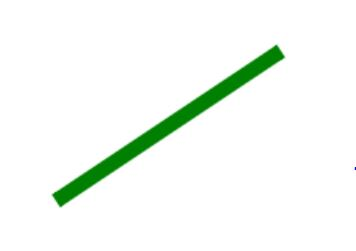### Stroke and fill

It is defined as stroke is used to create the outline and fill is used to create the color in the outline

``` <canvas id="mycanvas" height="300" width="600"></canvas>
<script>
var canvas = document.getElementById("mycanvas");
var context = canvas.getContext("2d");
context.beginPath();
context.moveTo(10, 10);
context.lineTo(10, 150);
context.lineTo(110, 50);
context.lineTo(10, 10);
context.stroke();
context.closePath();
</script>
```

Stroke

In the above code snippet we have defined the` stroke` by using the stroke().we have three line to functions to draw the triangle to create the outline of the stroke

output### Fill

It is used to` fill` the canvas with the color

```<canvas id="mycanvas" height="300" width="600"></canvas>
<script>
var canvas = document.getElementById("mycanvas");
var context = canvas.getContext("2d");
context.beginPath();
context.moveTo(10, 10);
context.lineTo(10, 150);
context.lineTo(110, 50);
context.lineTo(10, 10);
context.fill();
context.closePath();
</script>
```

Fill

In the above code snippet we have defined the` fill` which is used to create the color in the canvas

output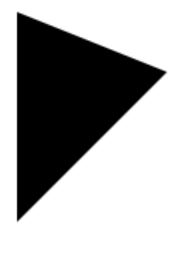### Line width

It is used to increase the width of the line

``` <canvas id="myCanvas" width="578" height="200"></canvas>
<script>
var canvas = document.getElementById('myCanvas');
var context = canvas.getContext('2d');

context.beginPath();
context.moveTo(10, 40);
context.lineTo(250, 50);
context.lineWidth = "10";
context.strokeStyle = "blue";
context.stroke();
</script>
```

Line width

In the above code snippet we have defined how to increase the` line width`, we have taken the line width as 10 to increase the line width and color as blue.

output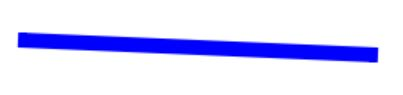### Line cap

It is used to create the line shapes by using round, butt and square

``` <canvas id="myCanvas" width="578" height="200"></canvas>
<script>
var canvas = document.getElementById('myCanvas');
var context = canvas.getContext('2d');

context.beginPath();
context.moveTo(200, canvas.height / 2 - 50);
context.lineTo(canvas.width - 100, canvas.height / 2 - 50);
context.lineWidth = 20;
context.strokeStyle = 'green';
context.lineCap = 'round';
context.stroke();

context.beginPath();
context.moveTo(500, canvas.height  - 50);
context.lineTo(canvas.width - 200, canvas.height / 2 - 50);
context.lineWidth = 15;
context.strokeStyle = 'pink';
context.lineCap = 'butt';
context.stroke();

context.beginPath();
context.moveTo(50, canvas.height / 2 - 50);
context.lineTo( 150, canvas.height / 2 - 50);
context.lineWidth = 10;
context.strokeStyle = 'blue';
context.lineCap = 'square';
context.stroke();
</script>
```

Line cap

In tha bove code snippet we have defined the line cap which defines the shapes of the lines as round , Butt and square. We have three lines to define the three different shapes of the lines

output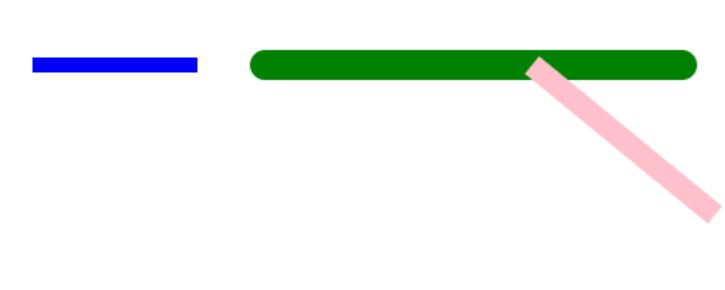### Line join:

It is used to join the lines by using bevel, miter and round properties

```    <canvas id="myCanvas" width="578" height="200"></canvas>
<script>
var c = document.getElementById("myCanvas");
var ctx = c.getContext("2d");
ctx.beginPath();
ctx.lineJoin = "miter";
ctx.moveTo(10, 150);
ctx.lineTo(100, 100);
ctx.lineTo(50, 16);
ctx.lineWidth = 15;
ctx.strokeStyle = "pink";
ctx.stroke();

ctx.beginPath();
ctx.lineJoin = "round";
ctx.moveTo(300, 120);
ctx.lineTo(200  , 180);
ctx.lineTo(150, 100);
ctx.linewidth = 15;
ctx.strokestyle = "pink";
ctx.stroke();

ctx.beginPath();
ctx.lineJoin = "bevel";
ctx.moveTo(400, 250);
ctx.lineTo(19, 180);
ctx.lineTo(130, 100);
ctx.linewidth = 15;
ctx.strokeStyle = "green";
ctx.stroke();
</script>
```

Linejoin

In the above code snippet we have defined three shapes of the linejoin() to display the corners as

• Bevel - It is used to display the shape of the corner as bevel
• Miter - It is used to display the shape of the corner as sharp
• Round - It is used to display the shape of the corner as rounded

outputArc

It is used to draw the arc

``` <canvas id="myCanvas" width="578" height="200"></canvas>
<script>
var c = document.getElementById("myCanvas");
var ctx = c.getContext("2d");
ctx.beginPath();
ctx.arc(10, 50, 15, 0, Math.PI);
ctx.stroke();
</script>
```

Arc

In tha bove code snippet we have defined how to create the `arc.` We have taken the ctx.arc() to define the arc by declaring the values of the arc

output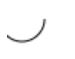It is used to draw the quadrature curves

```<canvas id="myCanvas" width="578" height="200"></canvas>
<script>
var c = document.getElementById("myCanvas");
var ctx = c.getContext("2d");
ctx.beginPath();
ctx.moveTo(20, 20);
ctx.stroke();
</script>
```

In the above code snippet we have defined the` quadrature curves` we have taken the var c as document.getelementbyid as ("mycanvas") and var ctx as c.getcontext("2d")

• ctx.beginpath() - It is used to start the path
• ctx.moveto -  It is used to place the x and y positions of the canvas
• ctx.quadrature curves - It is used to place the positions of the curves

output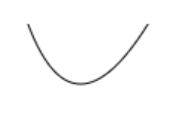### Beizer curveto()

It is used to add the point to create the bezier curve. The bezier curve uses two points such as beginpath and moveto()

``` <canvas id="myCanvas" width="578" height="200"></canvas>
<script>
var c = document.getElementById("myCanvas");
var ctx = c.getContext("2d");
ctx.beginPath();
ctx.moveTo(20, 40);
ctx.bezierCurveTo(20, 100, 200, 100, 200, 20);
ctx.stroke();
</script>
```

Beizer curveto

In the above code snippet we have defined the bezier curve. I n order to create the bezier curve we need to use the beginpath and moveto, We have moveto() as (x, y) and the bezier curve as ()required for the values.

output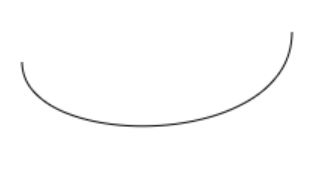Views: 3025 | Post Order: 45